# IB DP Chemistry: SL复习笔记4.2.2 Shapes of Molecules

### Shapes of Molecules

• When an atom forms a covalent bond with another atom, the electrons in the different bonds and the non-bonding electrons in the outer shell all behave as negatively charged clouds and repel each other
• In order to minimise this repulsion, all the outer shell electrons spread out as far apart in space as possible
• Molecular shapes and the angles between bonds can be predicted by the valence shell electron pair repulsion theory known by the abbreviation VSEPR theory
• VSEPR theory consists of three basic rules:
1. All electron pairs and all lone pairs arrange themselves as far apart in space as is possible.
2. Lone pairs repel more strongly than bonding pairs.
3. Multiple bonds behave like single bonds
• These three rules can be used to predict the shape of any covalent molecule or ion, and the angles between the bonds
• The regions of negative cloud charge are known as domains and can have one, two or three pairs electrons

#### Two electron domains

• If there are two electron domains on the central atom, the angle between the bonds is 180o
• Molecules which adopt this shape are said to be LINEAR
• Examples of linear molecules include BeCl2, CO2, and HC≡CH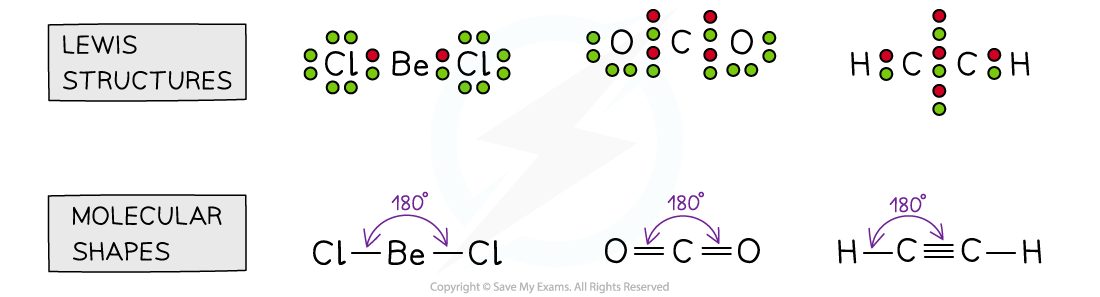Two electron domain molecules

#### Three electron domains

• If there are three electron domains on the central atom, the angle between the bonds is 120o
• Molecules which adopt this shape are said to be TRIANGULAR PLANAR or TRIGONAL PLANAR
• Examples of three electrons domains which are all bonding pairs include BFand CH2CHand CH2O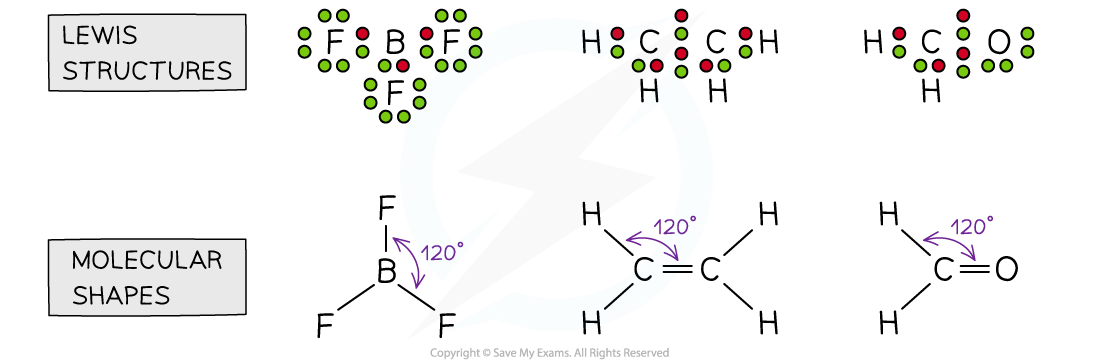Molecules with three electron domains

• If one of these electron domains is a lone pair, the bond angle is slightly less than 120o due to the stronger repulsion from lone pairs, forcing the bonding pairs closer together.
• E.g. SO2
• The bond angle is approximately = 118oThe shape of sulfur dioxide

• Sulfur dioxide is an example of a molecule that 'expands the octet' as you will see there are 10 electrons around the sulfur atom which is possible for 3rd period elements and above
• This shape is no longer called triangular planar as the shape names are only based on the atoms present, this molecule is BENT LINEAR

#### Four electron domains

• If there are four electron domains on the central atom, the angle between the bonds is approx 109o. E.g. CH4, NH4+
• Molecules which adopt this shape are said to be TETRAHEDRAL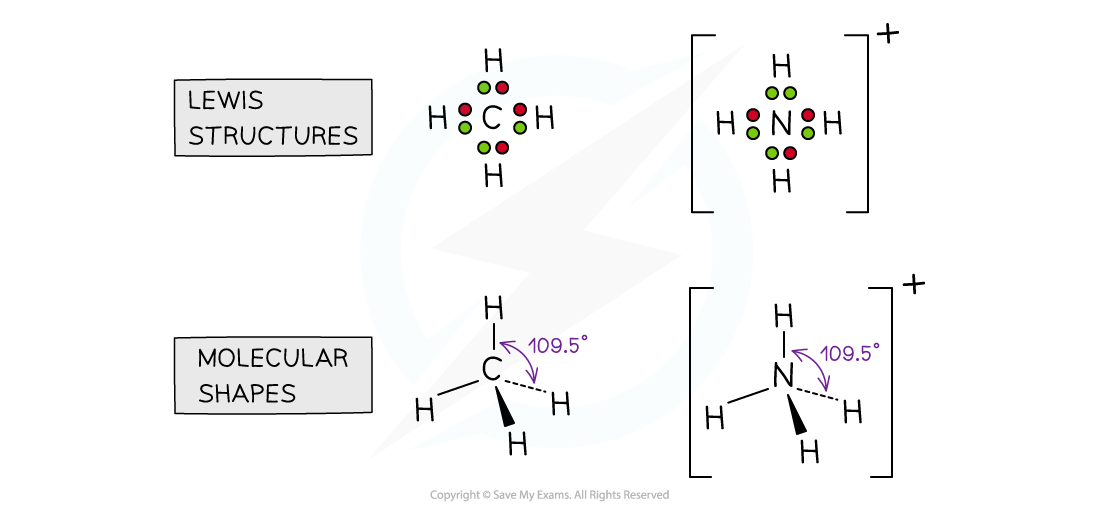Molecules with four electron domains

• If one of the electron domains is a lone pair, the bond angle is slightly less than 109o, due to the extra lone pair repulsion which pushes the bonds closer together (approx 107o). E.g. NH3,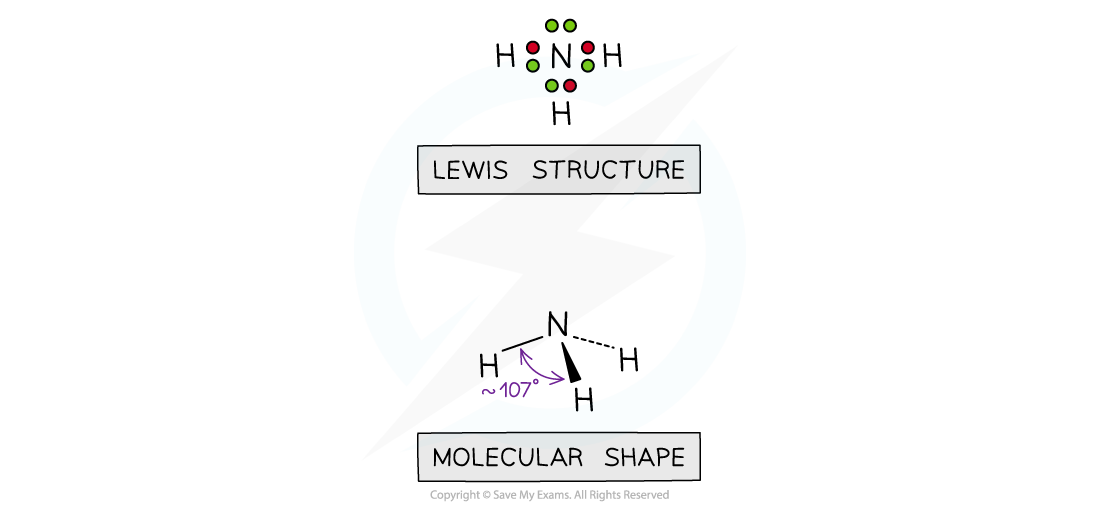The shape of ammonia

• Molecules which adopt this shape are said to be TRIANGULAR PYRAMIDAL or TRIGONAL PYRAMIDAL
• If two of the electron domains are lone pairs, the bond angle is also slightly less than 109o, due to the extra lone pair repulsion (approx 104o). E.g. H2O
• Molecules which adopt this shape are said to be BENT or ANGULAR or BENT LINEAR or V-shaped (when viewed upside down)The shape of water

• Lone pairs are pulled more closely to the central atoms so they exert a greater repulsive force than bonding pairs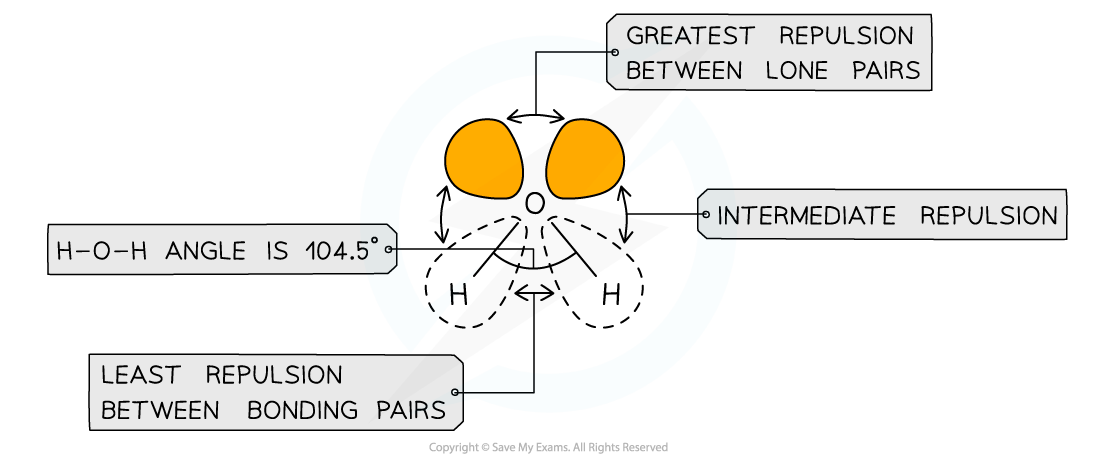Different types of electron pairs have different repulsive forces

Summary table of electron domains and molecular shapes

• These are the domains and molecular geometries you need to know for Standard Level: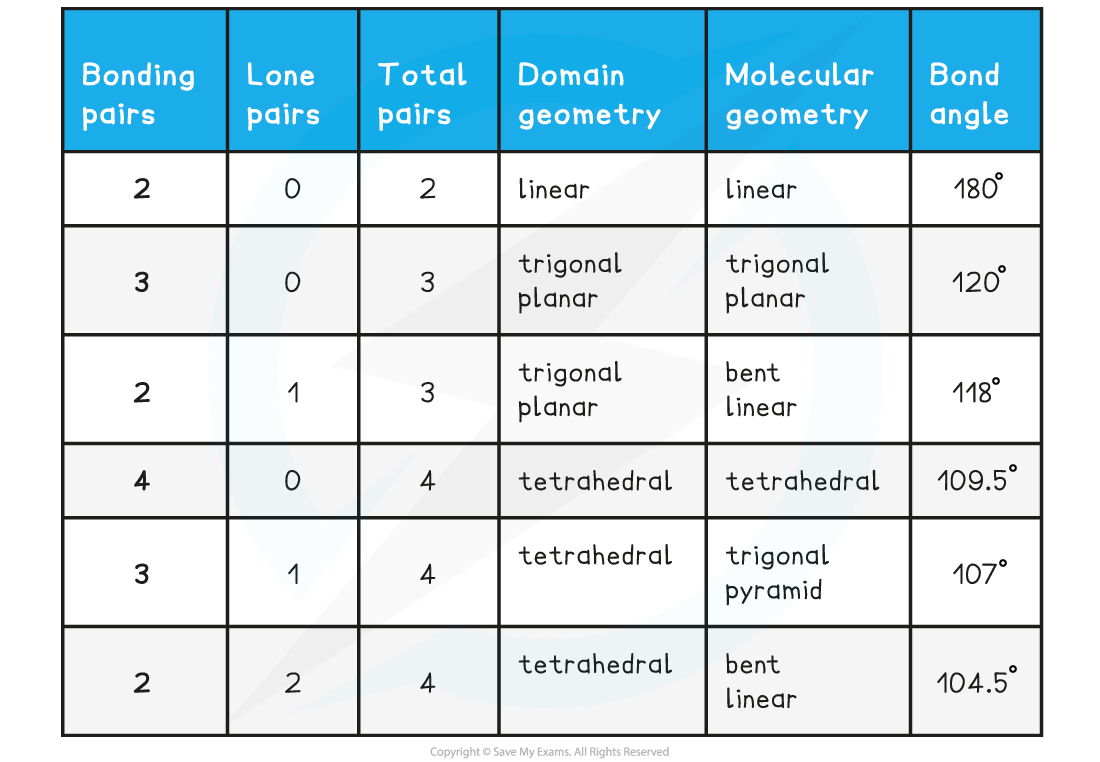#### Exam Tip

Be careful to distinguish between molecular shape and electron domain shape as it can be easy to confuse the two. Sometimes they are the same as is the case of methane, but other times they can be different like ammonia which has a tetrahedral domain shape, but triangular pyramid molecular shape. Always draw the Lewis structure before you attempt to deduce the shape and bond angle as you could easily miss some lone pairs